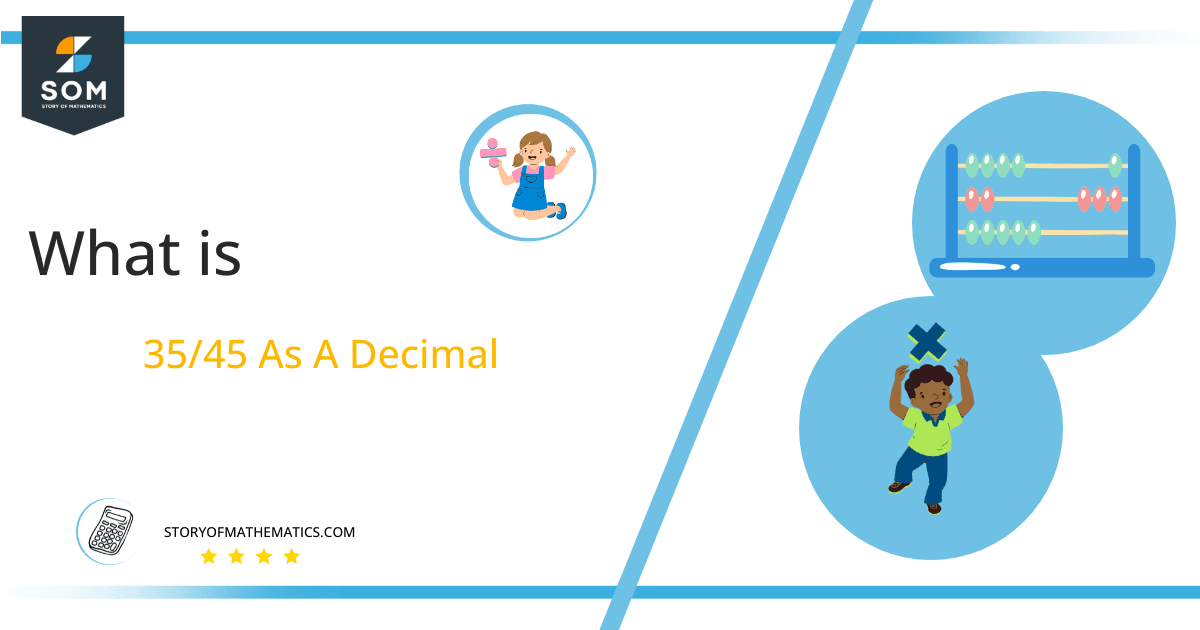# What Is 35/45 as a Decimal + Solution With Free Steps

The fraction 35/45 as a decimal is equal to 0.77777778.

A Decimal Number is a numerical quantity that has two parts a whole number value and the fractional value of that whole number. e.g. 1.5 is a decimal value where 1 is the whole number and 0.5 is the fractional value of 1.

Here, we are more interested in the division types that result in a Decimal value, as this can be expressed as a Fraction. We see fractions as a way of showing two numbers having the operation of Division between them that result in a value that lies between two Integers.Now, we introduce the method used to solve said fraction to decimal conversion, called Long Division, which we will discuss in detail moving forward. So, let’s go through the Solution of fraction 35/45.

## Solution

First, we convert the fraction components, i.e., the numerator and the denominator, and transform them into the division constituents, i.e., the Dividend and the Divisor, respectively.

This can be done as follows:

Dividend = 35

Divisor = 45

We introduce the most important quantity in our division process: the Quotient. The value represents the Solution to our division and can be expressed as having the following relationship with the Division constituents:

Quotient = Dividend $\div$ Divisor = 35 $\div$ 45

This is when we go through the Long Division solution to our problem. The following figure shows the long division:Figure 1

## 35/45 Long Division Method

We start solving a problem using the Long Division Method by first taking apart the division’s components and comparing them. As we have 35 and 45, we can see how 35 is Smaller than 45, and to solve this division, we require that 35 be Bigger than 45.

This is done by multiplying the dividend by 10 and checking whether it is bigger than the divisor or not. If so, we calculate the Multiple of the divisor closest to the dividend and subtract it from the Dividend. This produces the Remainder, which we then use as the dividend later.

Now, we begin solving for our dividend 35, which after getting multiplied by 10 becomes 350.

We take this 350 and divide it by 45; this can be done as follows:

350 $\div$ 45 $\approx$ 7

Where:

45 x 7 = 315

This will lead to the generation of a Remainder equal to 350 – 315 = 35. Now this means we have to repeat the process by Converting the 35 into 350 and solving for that:

350 $\div$ 45 $\approx$ 7

Where:

45 x 7 = 315

This, therefore, produces another Remainder equal to 350 – 315 = 35. Now we must solve this problem to Third Decimal Place for accuracy, so we repeat the process with dividend 350.

350 $\div$ 45 $\approx$ 7

Where:

45 x 7 = 315

Finally, we have a Quotient generated after combining the three pieces of it as 0.777=z, with a Remainder equal to 35.Images/mathematical drawings are created with GeoGebra.